Function Repository Resource:

# DirectionalD

Compute the directional derivative of a function

Contributed by: Wolfram Staff (original content by Alfred Gray)
 ResourceFunction["DirectionalD"][f,r,vars] computes the derivative of a function f in the direction r with variables vars.

## Details and Options

The directional derivative of a function f at the point p along an arbitrary vector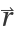represents the instantaneous rate of change of the function at that direction.
ResourceFunction["DirectionalD"] is a generalization of a partial derivative in which the rate of change is taken with respect to one of the variables, considering the rest as constant.
The directional derivative is formally defined as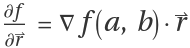, whereis the vector that indicates the direction.
An alternative definition of the directional derivative is taken to be with respect to a normalized arbitrary nonzero vector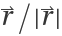.
Other notations for the directional derivative are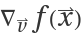,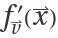,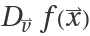,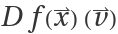,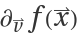,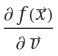,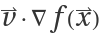and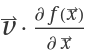.

## Examples

### Basic Examples (2)

Directional derivative of a function of two variables:

 In:=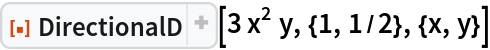Out=Directional derivative of a function of three variables:

 In:=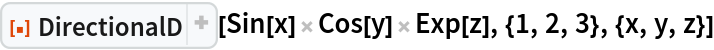Out=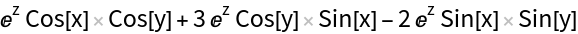### Scope (6)

Directional derivative with a zero component in one direction:

 In:=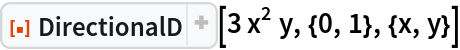Out=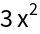A unit vector along direction (3/2,1):

 In:=Out=Without normalization:

 In:=Out=Evaluated at the point p=(3/2,1):

 In:=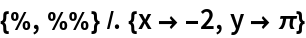Out=Define a function:

 In:=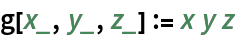Compute the directional derivative:

 In:=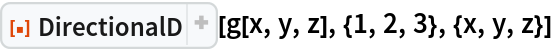Out=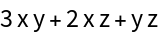Directional derivative in the direction (-1,-1/2) evaluated at the point (-1,3/2):

 In:=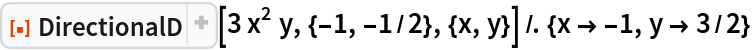Out=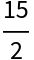A plot of the directional derivative:

 In:=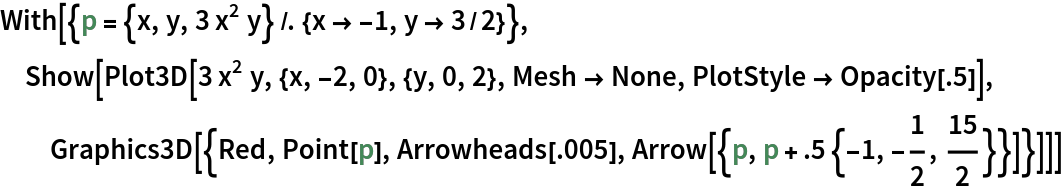Out=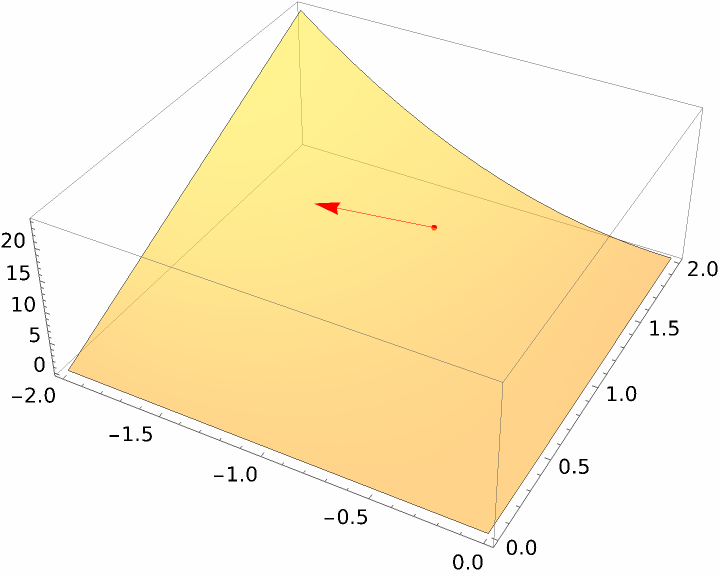Visualize directional derivatives over a surface:

 In:=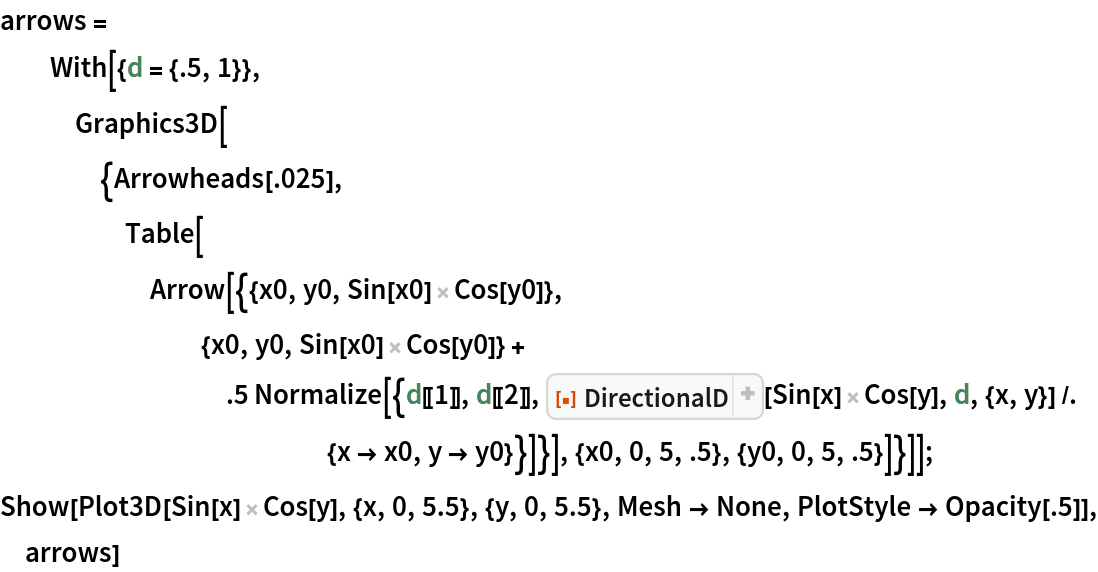Out=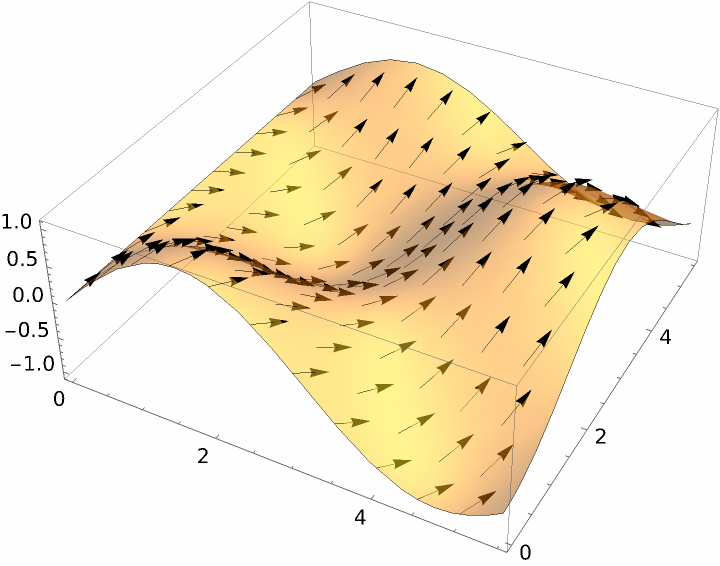The directional derivative (red) and the gradient (blue):

 In:=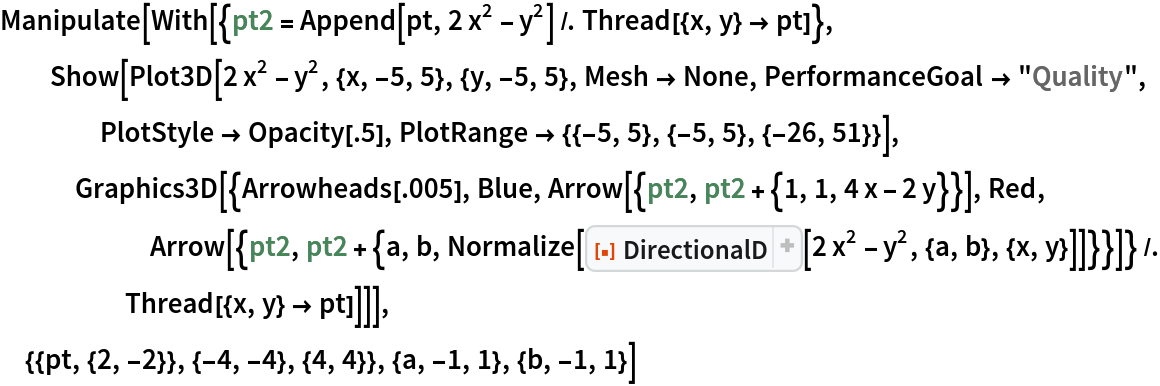Out=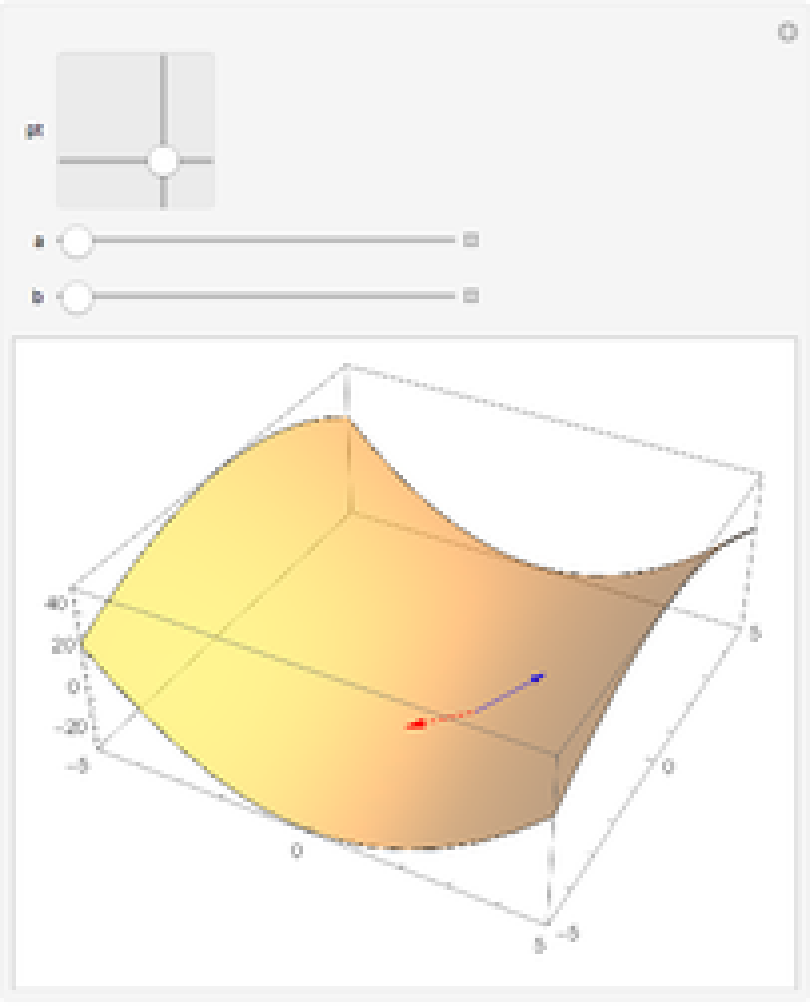Enrique Zeleny

## Version History

• 2.0.0 – 01 October 2020
• 1.0.0 – 15 June 2020